ODETLAP Tiling

by Dan Tracy

April 26, 2007

Given a matrix of terrain elevations, we can easily render it as an image in OpenGL. The simplest approach is to use GL_TRIANGLES to linearly interpolate the space between the grid points. However, this results in a very blocky image. We could generate a smoother terrain by adding more grid points and interpolating them with a nonlinear method. One such method is ODETLAP (http://www.ecse.rpi.edu/Homepages/wrf/pmwiki/Research/AlternateTerrainReps#toc16), developed by W.R. Franklin. However, the current implementation does not work efficiently on grids larger than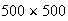. When solving for an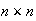grid of points, the space complexity is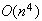, and the time complexity is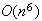. We would like to do better than this.

The original ODETLAP formulation:

Given agrid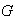, where some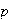grid points have known values, and the rest (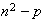grid points) are unknown. We can transform the matrixinto a vector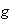of size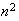. We produce a system of linear equations by setting the value of each point equal to the average of its four neighbors (or fewer than four for boundary points). Then additional equations are produced by setting each known grid point equal to its known value. This results in an overdetermined system of linear equations. There are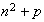equations forunknowns.

The known points are allowed to change in order to produce a smoother result. The first set of equations is multiplied a smoothness parameter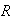. This specifies the relative weight between the first set of equations, which enforce the smoothness of the solution, and the second set of equations, which enforce the accuracy of the known points. For values ofclose to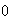, the values of the known points will stay relatively fixed. For larger values of, the known points may drift in order to produce a smoother solution. Asapproaches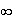, the solution approaches a flat plane.

The system of equations can be expressed as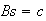, whereis of size, and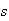and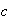are each of size. This system was solved with some MATLAB code, which I obtained from Zhongyie Xie.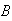is formed with MATLAB's sparse data structure, so its storage requirement is only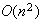. However, solving the matrix equation will still requirestorage.

I eliminated the smoothness parameter and the second set of equations by absorbing them into the first set of equations. The known points are no longer treated as variables. In the equations, the variables for the known points are replaced by their known constants. I now haveequations forunknowns. The solution of this system corresponds to the solution of the original ODETLAP algorithm asapproaches.

Next I focused on a special case: Given anterrain of elevations, with every grid point known, expand the terrain to a higher resolution (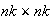) by insertingunknown points between each of the known points. This new terrain can then be partitioned intosubgrids of size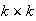so that the four corners of each subgrid are known. Each known point would belong to four subgrids (except the boundary points). Each subgrid can then be solved separately, and their solutions stitched together afterwards. For each point that belongs to more than one subgrid, the solutions are averaged together. In this manner, we can avoid the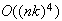space complexity that the original ODETLAP incurs. However, this produces a less smooth solution than the original ODETLAP.

A further approach is to use subgrids of size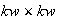. The number of subgrids is still, so there is considerable overlap between the subgrids. Like before, for each point that belongs to more than one subgrid, the solutions are averaged together. This produces a smoother solution, though with a higher cost.

Now we must measure the tradeoff of efficiency vs. accuracy of the new methods. Efficiency can be measured with cpu time. For accuracy, we can compute the solution (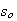) with the original ODETLAP as well as the solution (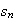) with the new method. The error vector is then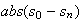. From this, I extracted the maximum and the average error. However, there may be large examples where we can compute the solution with the new method, but it is not feasible to compute the solution with the original ODETLAP. In this case, we can examine the residual of the overdetermined system instead: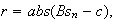whereandcorrespond to the equations generated by the original ODETLAP. However, there does not usually exist a solution such that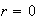, so it is more difficult to determine how close we are to the best solution. But we can compare competing methods against each other this way. Also, this residual corresponds to the "sharpness" of the solution in terms of the elevation units of the original data.

An iterative scheme of solving the overdetermined system of equations could be greatly beneficial. Using the previous method, we can make a very good initial guess into the algorithm. Using the notation thatis the computed solution, and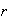is the residual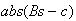, we should be able to compute the Jacobian matrix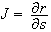. The original ODETLAP will give us the solution that minimizes the residual, so thatfor every. This could be used in a scheme like Newton's method, but I have not been able to complete the algorithm.

In the numerical experiments, I used theupper-left corner of the Lake Champlain data set. Then I ran the ODETLAP to produce a higher resolutionterrain. The values chosen for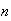and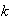are reported in the table. I compared the results from different values of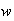, the size of the subgrids, against the results from the original ODETLAP. The tables are at the end of the paper.

The residuals are quite large when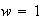, though not unreasonable. However, the results start to look very good at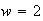. That is, the residual fromis on the same order of magnitude as the residual from the original ODETLAP. And there is considerable speedup. For example, for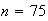,, the original ODETLAP takes 233 seconds, and themethod takes 11.5 seconds, while the maximum residual only increases from 1.89 to 2.07, and the average residual only increases from 0.0865 to 0.0875. When rendered, these differnce should hardly be noticeable. We now have a very efficient and practical interpolative scheme.

Tiling demonstration

The intersections of the solid blue lines are the known points. The intersections of the dotted blue lines are the unknown points. The red boxes are the tiles that ODETLAP is performed on.I downsampled the Lake Champlain data to 100×100:Then I expanded it to a 400×400 grid using my tiled ODETLAP scheme:The tiled scheme took 23 seconds to compute. The original untiled scheme took 15 minutes to compute.

References:

http://www.ecse.rpi.edu/Homepages/wrf/pmwiki/Research/AlternateTerrainReps#toc16

Numerical results:

n=15, k=6
Method    Time    Max_Error  Avg_Error  Max_Residual Avg_Residual
orig   2.48e+000  0.00e+000  0.00e+000   8.77e-001    5.52e-002
w=1   1.48e+000  2.91e+000  3.66e-001   1.26e+000    7.36e-002
w=2   2.02e+000  1.37e+000  1.56e-001   1.05e+000    5.83e-002
w=3   3.39e+000  9.12e-001  1.20e-001   1.03e+000    5.81e-002
w=4   5.47e+000  9.41e-001  1.08e-001   1.03e+000    5.83e-002
w=5   8.27e+000  9.30e-001  1.02e-001   1.03e+000    5.90e-002
w=6   1.03e+001  9.31e-001  1.01e-001   1.03e+000    5.96e-002
w=7   1.29e+001  9.31e-001  1.05e-001   1.03e+000    6.00e-002
w=8   1.64e+001  9.31e-001  1.11e-001   1.03e+000    6.01e-002
w=9   1.59e+001  9.31e-001  1.01e-001   1.03e+000    5.97e-002
w=10   1.66e+001  9.31e-001  8.99e-002   1.03e+000    5.90e-002

n=20, k=6
Method    Time    Max_Error  Avg_Error  Max_Residual Avg_Residual
orig   6.88e+000  0.00e+000  0.00e+000   7.28e-001    4.47e-002
w= 1   2.70e+000  2.75e+000  3.06e-001   1.26e+000    6.05e-002
w= 2   3.91e+000  1.05e+000  1.29e-001   8.78e-001    4.72e-002
w= 3   6.88e+000  9.12e-001  1.03e-001   8.37e-001    4.72e-002
w= 4   1.27e+001  7.81e-001  8.96e-002   8.07e-001    4.73e-002
w= 5   1.90e+001  8.21e-001  8.44e-002   7.92e-001    4.77e-002
w= 6   2.84e+001  8.16e-001  8.37e-002   7.81e-001    4.82e-002
w= 7   3.36e+001  8.16e-001  8.42e-002   8.02e-001    4.83e-002
w= 8   4.82e+001  8.16e-001  8.58e-002   7.87e-001    4.84e-002

n=30, k=4
Method    Time    Max_Error  Avg_Error  Max_Residual Avg_Residual
orig   1.20e+001  0.00e+000  0.00e+000   1.51e+000    8.75e-002
w= 1   5.66e+000  2.64e+000  2.45e-001   2.13e+000    9.74e-002
w= 2   6.81e+000  1.04e+000  1.03e-001   1.59e+000    8.89e-002
w= 3   9.61e+000  7.91e-001  7.94e-002   1.52e+000    8.88e-002
w= 4   1.35e+001  6.75e-001  6.65e-002   1.51e+000    8.89e-002
w= 5   2.00e+001  6.92e-001  6.13e-002   1.51e+000    8.95e-002
w= 6   2.89e+001  6.88e-001  5.93e-002   1.51e+000    8.98e-002
w= 7   3.93e+001  6.88e-001  5.95e-002   1.51e+000    9.01e-002
w= 8   5.14e+001  6.88e-001  6.08e-002   1.51e+000    9.02e-002

new machine:

n=50, k=4
Method  Time    Max_Error  Avg_Error  Max_Residual Avg_Residual
orig   4.71e+01  0.00e+00  0.00e+00   1.89e+00    9.08e-02
w= 1   2.36e+00  3.58e+00  2.63e-01   3.09e+00    1.01e-01
w= 2   4.98e+00  1.67e+00  1.13e-01   2.07e+00    9.18e-02
w= 3   9.90e+00  1.14e+00  8.26e-02   2.05e+00    9.13e-02
w= 4   1.60e+01  1.11e+00  6.70e-02   2.00e+00    9.13e-02
w= 5   2.57e+01  1.11e+00  5.97e-02   1.98e+00    9.16e-02
w= 6   3.97e+01  1.10e+00  5.58e-02   1.97e+00    9.18e-02
w= 7   6.14e+01  1.10e+00  5.33e-02   1.96e+00    9.19e-02

n=60, k=4
Method  Time    Max_Error  Avg_Error  Max_Residual Avg_Residual
orig   8.83e+01  0.00e+00  0.00e+00   1.89e+00    9.21e-02
w= 1   3.63e+00  4.09e+00  2.72e-01   3.09e+00    1.03e-01
w= 2   8.05e+00  1.83e+00  1.17e-01   2.07e+00    9.33e-02
w= 3   1.33e+01  1.22e+00  8.32e-02   2.05e+00    9.27e-02
w= 4   2.15e+01  1.11e+00  6.53e-02   2.00e+00    9.26e-02
w= 5   3.85e+01  1.11e+00  5.66e-02   1.98e+00    9.29e-02
w= 6   5.96e+01  1.10e+00  5.19e-02   1.97e+00    9.31e-02

n=75, k=4
Method  Time    Max_Error  Avg_Error  Max_Residual Avg_Residual
orig   2.33e+02  0.00e+00  0.00e+00   1.89e+00    8.65e-02
w= 1   6.25e+00  4.09e+00  2.56e-01   3.09e+00    9.70e-02
w= 2   1.15e+01  1.83e+00  1.11e-01   2.07e+00    8.75e-02
w= 3   1.88e+01  1.27e+00  7.86e-02   2.05e+00    8.68e-02
w= 4   3.43e+01  1.18e+00  6.16e-02   2.00e+00    8.68e-02
w= 5   6.16e+01  1.14e+00  5.32e-02   1.98e+00    8.70e-02
w= 6   9.62e+01  1.11e+00  4.83e-02   1.97e+00    8.72e-02
w= 7   1.51e+02  1.10e+00  4.51e-02   1.96e+00    8.73e-02

n=85, k=4
Method    Time    Max_Error  Avg_Error  Max_Residual Avg_Residual
orig   3.64e+02  0.00e+00  0.00e+00   1.89e+00    8.74e-02
w= 1   8.78e+00  4.09e+00  2.58e-01   3.09e+00    9.84e-02
w= 2   1.27e+01  1.83e+00  1.12e-01   2.07e+00    8.82e-02
w= 3   2.43e+01  1.22e+00  7.83e-02   2.05e+00    8.74e-02
w= 4   4.47e+01  1.11e+00  6.07e-02   2.00e+00    8.73e-02
w= 5   8.20e+01  1.11e+00  5.11e-02   1.98e+00    8.75e-02